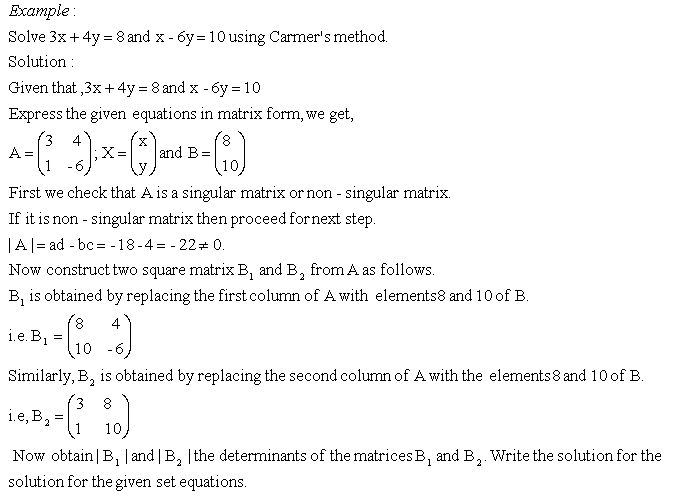Email us to get an instant 20% discount on highly effective K-12 Math & English kwizNET Programs!

Online Quiz (WorksheetABCD)

Questions Per Quiz = 2 4 6 8 10

High School Mathematics - 26.5 Solving Linear Equations Using Cramer's Method

 Cramer's Rule: You can use determinants to solve a system of linear equations. The method is called Cramer's rule and is named after the Swiss mathematician Gabriel Cramer. It uses the coefficient matrix of the linear system.Directions: Solve the following system using Cramer's rule. Also write at least five examples of your own.
 Q 1: Solve 4x + 5y = 50 and 3x+ 2y = 27 using Cramer's method.x = 5 and y = 4x = 5 and y = 7x = 5 and y = 6x = 4 and y = 6 Q 2: Solve 2x - 5y = 11 and 3x + 4y = 5 using Cramer's method.x = 3 and y = -1x = 3 and y = 1x = 2 and y = -3x = -3 and y = -1 Q 3: Solve 2x + 4y = 10 and 3x + 2y =7 using Cramer's method.x = 2 and y = 4x = 1 and y = 2x = 1 and y = 3x = 2 and y = 3 Q 4: Solve 2x + 3y = 13 and 3x + y = 9 using Cramer's method.x = 4 and y = 2x = 2 and y = 5x = 2 and y = 3x = 2 and y = 4 Q 5: Solve 2x + 5y = 16 and 3x + 4y = 17 using Cramer's method.x = 5 and y = 2x = 3 and y = 2x = 5 and y = 4x = 3 and y = 5 Q 6: Solve 2x+ 5y = 26 and 3x + 7y = 37 using Cramer's method.x = 5 and y = 4x = 2 and y = 4x = 3 and y = 2x = 3 and y = 4 Question 7: This question is available to subscribers only! Question 8: This question is available to subscribers only!